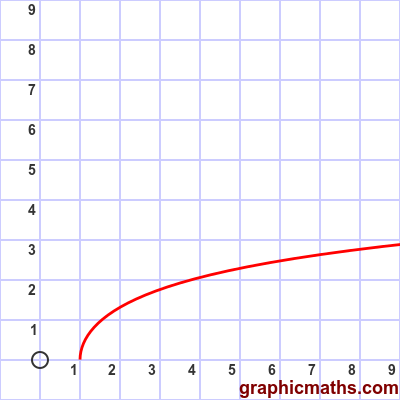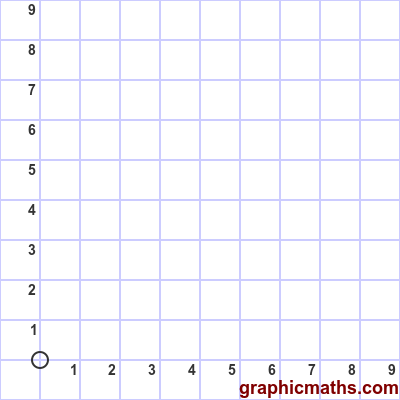# arcosh function

Martin McBride, 2020-02-03
Tags arcosh
Categories hyperbolic functions

The arcosh function is a hyperbolic function. It is the inverse of the cosh, and is also known as the inverse hyperbolic cosine function.

## Equation and graph

The arcosh function is defined as the inverse of cosh, ie if:

$$x = \cosh y$$

then:

$$y = \operatorname{arcosh} x$$

There is a also a formula for finding arcosh directly:

$$\operatorname{arcosh} x= \ln{x+{\sqrt {x^{2}-1}}}$$

Here is a graph of the function:The function is valid for x >= 1.

## arcosh as inverse of cosh

This animation illustrates the relationship between the cosh function and the arcosh function:The first, blue, curve is the cosh function.

The grey dashed line is the line $y=x$.

The second, red, curve is the arcosh function. As with any inverse function, it is identical to the original function reflected in the line $y=x$.